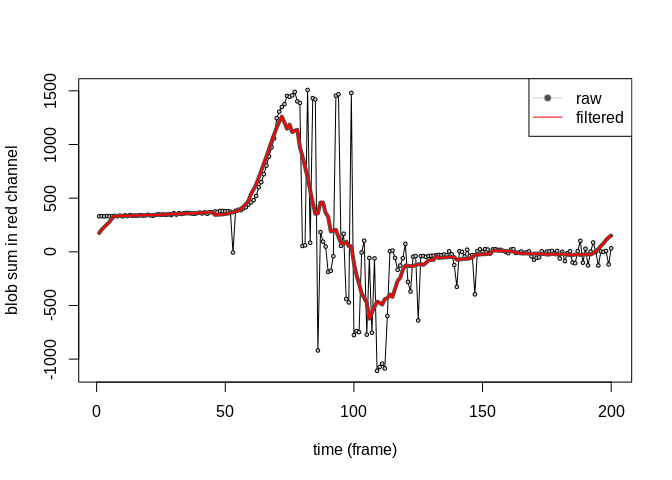# Analyze Volcano Video Data with R

The motivation for the imagefx package was to better use volcano video data as a monitoring tool. This tutorial demonstrates how to characterize a variety of eruptive styles including plume emissions and lava lake activity by synthesizing images into time series signals. These signals may further be analyzed for monitoring purposes or be used as inputs into machine learning applications.

The tutorial is broken into the following sections:

We assume the user has R installed on their computer and has a basic familiarity with R syntax, base functions, and coding in general. To install the imagefx package from the R console use install.packages('imagefx') and then load with:

library(imagefx)

Data files can have various formats including jpg, png, tiff, etc… and it is important that appropriate packages are installed to read in the desired data set. For example, if you are trying to read in images with the extension .jpeg, you will need to install the jpeg package using

install.packages('jpeg')

Image data should be saved within a well organized directory. This will ease reading in and analyzing sequences of images (i.e. video), which is discussed later. In the case of webcam data, raw image frames may be located in /name_of_camera/year/month/day/hour/. Please keep in mind the difference in directory nomenclature between Apple, Windows, and Linux operating systems.

The below code is an example of how to read in an image file (in this case a .jpeg) once the jpeg package is installed.

library(jpeg)

## identify where the image files are located
img.dir <- '/name_of_camera/year/month/day/hour/'

## which image will you read in
img.file <- 'sakurajima.jpeg'

## read in the image using functions from the jpeg package
## note the image 'sakurajima' comes preloaded with the imagefx package
sakurajima <- readJPEG(paste(img.dir,img.file,sep=""))

Once the data are loaded in the R workspace, you can get a sense for the structure and the image itself with:

## what are the dimensions of the image
dim(sakurajima)
#>  300 400   3

## plot the image using the imagefx package
image2(sakurajima,asp=1,xlab="image rows",ylab="image columns")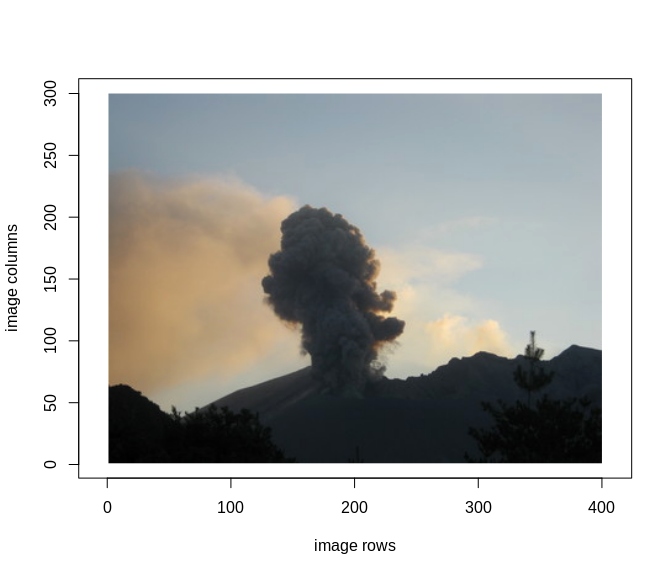In this case the dimensions of the image correspond to the number of rows, number of columns, and number of color channels, respectively. In other words, this image has 300 rows, 400 columns and a red, green, and blue color channel. If the image your read in is gray scaled, it is likely the image will be read in as a matrix with only rows and columns.

Note the origin in the image is the bottom left corner and that the 400 rows correspond to the width of the image and the 300 columns correspond to the height of the image. This is an important, and sometimes confusing, aspect of how R reads in image data and should be considered when cropping and further processing the image.

## Preprocess image

Before analyzing volcano image data, preprocessing is helpful, and sometimes necessary, to highlight the plume region. To this end, we first crop the image and then remove an color trends not associated with volcanic activity.

#### Crop region above the vent

We are interested in monitoring the plume activity above the crater rim and therefore must crop the image in one of two ways. If you do not already know the bottom left and top right corners of the crop region you can pick them manually by supplying the name of the image as the single input into the crop.image function:

## manually crop the image by clicking on the plot region
crop.manual <- crop.image(sakurajima)

The image will be plotted and the user must first select the bottom left corner then, the top right corner. The crop area will be indicated by a dashed rectangle and if deemed sufficient, the user may select crop in the top right margin of the plot. If the crop region needs to be modified, the user may click repick in the top left margin and repeat the picking process.

If you know the bottom left and top right corners of the crop region, you may crop the image automatically as such:

## define the corners of the crop region
xleft = 1
xright = 188
ybottom = 1
ytop = 396

## use the above points to automatically crop the image
crop.auto <- crop.image(sakurajima,xleft,ybottom,xright,ytop)

The output from crop.image is a list of length two whose first component is the cropped image and second is a vector indicating the locations of the crop region’s bottom left and top right corners in the original image. We can disregard the crop locations and keep the crop image and plot the results with:

## isolate the cropped image
img.crop <- crop.auto$img.crop ## Plot original and cropped images plot.new() split.screen(c(1,2)) #>  1 2 screen(1) image2(sakurajima,asp=1,xlab="",ylab="", main='Original') screen(2) image2(img.crop,asp=1,ylab="",xlab="", main='Cropped')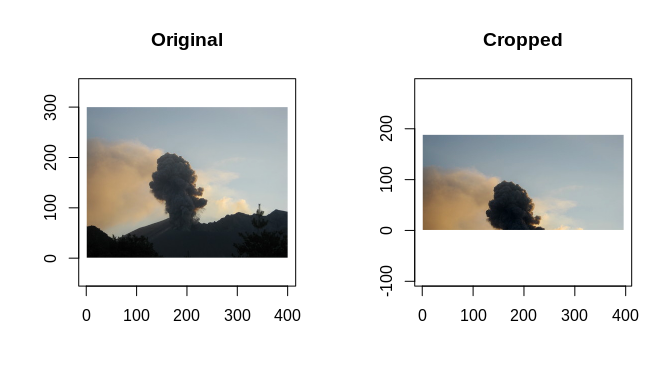## Perform blob detection We now find the most prominent color region in the processed image using a Laplacian of the Gaussian (LoG) blob detection algorithm. There are three inputs into the algorithm, which include a Gaussian filter mask, a point contained within the blob, and the window size used in a connected component algorithm. The Gaussian filter should have dimensions that match the image being analyzed and have a width (sigma) that best matches commonly observed plume activity. We assume the blob region will contain the pixel location associated with the value that deviates most from the mean. As an aside, removing the planar trend during the preprocessing is critical to finding this point. ## define a sigma value for the Gaussian filter sig=25 ## build a Gaussian mask based on this sigma and whose dimensions match the image gaus <- build.gaus(xdim=nrow(img.r.dtrend),ydim=ncol(img.r.dtrend),sig.x=sig) ## find the pixel value location in each channel that deviates most from the mean. max.r = which(abs(img.r.dtrend)==max(abs(img.r.dtrend)),arr.ind=TRUE) max.g = which(abs(img.g.dtrend)==max(abs(img.g.dtrend)),arr.ind=TRUE) max.b = which(abs(img.b.dtrend)==max(abs(img.b.dtrend)),arr.ind=TRUE) ## define a window size used in the connected component algorithm win.size=0.05 ## extract the blob from each channel blob.r <- blob.extract(img.r.dtrend,max.r,win.size,gaus) blob.g <- blob.extract(img.g.dtrend,max.g,win.size,gaus) blob.b <- blob.extract(img.b.dtrend,max.b,win.size,gaus) ################ #-- PLOTTING --# ################ ## note the blob points (blob$xy.coords) must be adjusted according to
## where the origin (0,0) is located in R image plots
blob.coords.r  <- blob.r$xy.coords blob.coords.r[,1] <- blob.r$xy.coords[,2]
blob.coords.r[,2] <- (blob.r$xy.coords[,1]-nrow(img.r))*-1 blob.coords.g <- blob.g$xy.coords
blob.coords.g[,1] <- blob.g$xy.coords[,2] blob.coords.g[,2] <- (blob.g$xy.coords[,1]-nrow(img.g))*-1

blob.coords.b  <- blob.b$xy.coords blob.coords.b[,1] <- blob.b$xy.coords[,2]
blob.coords.b[,2] <- (blob.b$xy.coords[,1]-nrow(img.b))*-1 close.screen(all.screens=TRUE) split.screen(c(1,3)) #>  1 2 3 screen(1) par(mar=c(0,0,2,0)) image2(img.r,asp=1,axes=FALSE) points(blob.coords.r,col=rgb(1,0,0,alpha=0.05),pch=16,cex=0.3) title('Red Channel',line=0,font=2,col='red',cex=2) screen(2) par(mar=c(0,0,2,0)) image2(img.g,asp=1,axes=FALSE) points(blob.coords.g,col=rgb(0,1,0,alpha=0.05),pch=16,cex=0.3) title('Green Channel',line=0,font=2,col='darkgreen',cex=2) screen(3) par(mar=c(0,0,2,0)) image2(img.b,asp=1,axes=FALSE) points(blob.coords.b,col=rgb(0,0,1,alpha=0.05),pch=16,cex=0.3) title('Blue Channel',line=0,font=2,col='darkblue',cex=2)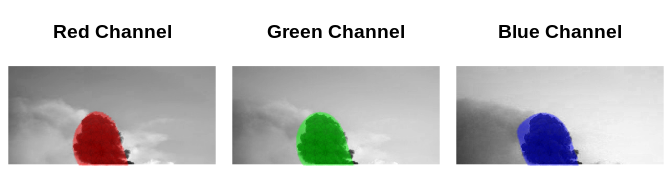## Calculate blob statistics The blob region is analyzed in terms of its color and spatial statistics. The color statistics include the sum, mean, standard deviation, skewness, and kurtosis of the blob region within image. Spatial statistics include the blob’s area as well as the centroid, standard deviation, skewness, and kurtosis of the blob’s position in the x and y directions. ## calculate the blob statistics in each RGB channel blob.stats.r <- calc.blob.stats(img.r.dtrend, blob.r$xy.coords)
blob.stats.g <- calc.blob.stats(img.g.dtrend, blob.g$xy.coords) blob.stats.b <- calc.blob.stats(img.b.dtrend, blob.b$xy.coords)

print(blob.stats.r)
#>       col_sum      col_mean        col_sd      col_skew      col_kurt
#> -2.735819e+03 -3.363849e-01  1.707969e-01  1.739634e+00  4.870199e+00
#>     blob_area        cent_x        cent_y      pos_sd_x      pos_sd_y
#>  8.133000e+03  1.448631e+02  1.726859e+02  2.712564e+01  2.517825e+01
#>    pos_skew_x    pos_skew_y    pos_kurt_x    pos_kurt_y
#> -2.038622e-01  7.504732e-02  1.961819e+00  2.028401e+00
print(blob.stats.g)
#>       col_sum      col_mean        col_sd      col_skew      col_kurt
#> -2457.9144759    -0.2902249     0.1531402     1.6930934     4.4913035
#>     blob_area        cent_x        cent_y      pos_sd_x      pos_sd_y
#>  8469.0000000   144.5105089   172.7800803    26.8902185    26.3916302
#>    pos_skew_x    pos_skew_y    pos_kurt_x    pos_kurt_y
#>    -0.1719626     0.0867709     1.9436651     2.0093046
print(blob.stats.b)
#>       col_sum      col_mean        col_sd      col_skew      col_kurt
#> -2.068136e+03 -2.336085e-01  1.180375e-01  1.629473e+00  4.442890e+00
#>     blob_area        cent_x        cent_y      pos_sd_x      pos_sd_y
#>  8.853000e+03  1.438746e+02  1.721113e+02  2.658124e+01  2.797816e+01
#>    pos_skew_x    pos_skew_y    pos_kurt_x    pos_kurt_y
#> -1.040236e-01  8.110596e-02  1.910216e+00  2.009131e+00

## Run algorithm on series of images

As mentioned earlier, a well organized directory is critical for proper time series analysis of images. Directories in which images are stored should be named in a way so that files are sorted with the oldest listed first and the newest last. For example, file names may be specific and include the hour, min, and second in the file name (e.g. 010101.jpeg) or, if the sampling rate is known, as a general numeric sequence with zero padding (e.g. 0001.jpeg)

## name the directory which holds the image files
img.dir <- 'raw_images/year/month/day/hour/'

## list the files in this directory.  Note they should be sorted from oldest to newest
img.files <- list.files(img.dir)

## how many statistics will you save.
## this will change if you modify the calc.blob.stats function
num.stats=14

## set up matrices to hold all the blob statistics for each RGB channel
all.blob.stats.r = matrix(NA,nrow=length(img.files),ncol=num.stats)
all.blob.stats.g = matrix(NA,nrow=length(img.files),ncol=num.stats)
all.blob.stats.b = matrix(NA,nrow=length(img.files),ncol=num.stats)

## loop over each image and perform the steps outlined above
i=1
while(i<=length(img.files)) {

## what is the current image file
cur.img.file <- paste(img.dir,img.files[i],sep="")

## read in the current image

## Preprocess the image ...
## Perform blob detection ...
## Calculate blob statistics ...

## save the statistics from the current frame to the all blob statistics matrix
all.blob.stats.r[i,] <- blob.stats.r
all.blob.stats.g[i,] <- blob.stats.g
all.blob.stats.b[i,] <- blob.stats.b

## move onto the next file
i=i+1
}

## combine the blob statistis associated with each RGB channel into a list
all.stats <- list(r=all.blob.stats.r, g=all.blob.stats.g, b=all.blob.stats.b)

## save all the statistics in an appropriate directory
save(all.stats,file='blob_stats/year/month/day/hour/blob_stats.RData')

We perform the outlined routine above on a series of image frames collected at Erebus Volcano, Antarctica in order to track eruptive activity through time. These data come preloaded with imagefx and show a single bubble burst from the lava lake.

## pick a single statistic from all the blob statistics
## in this case, the sum of the red channel blob region
blob.sum <- blob.stats$r[,1] ## PLOTTING ## ## set up the plot close.screen(all.screens=TRUE) split.screen(c(2,1)) #>  1 2 split.screen(c(1,3),screen=1) #>  3 4 5 ## plot some example images screen(3) par(mar=c(0,0,0,0)) image2(erebus.40,axes=FALSE,xlab="",ylab="",asp=1) text(40,nrow(erebus.40),labels='t1',pos=1,font=2,col='white') screen(4) par(mar=c(0,0,0,0)) image2(erebus.70,axes=FALSE,xlab="",ylab="",asp=1) text(40,nrow(erebus.70),labels='t2',pos=1,font=2,col='white') screen(5) par(mar=c(0,0,0,0)) image2(erebus.90,axes=FALSE,xlab="",ylab="",asp=1) text(40,nrow(erebus.90),labels='t3',pos=1,font=2,col='white') ## plot raw blob stats screen(2) plot(blob.sum,type='o',bg='gray80',xlab="",ylab='', pch=21, cex=0.5, ylim=c(min(blob.sum),max(blob.sum)+500),axes=FALSE) ## add a title title(main='Blob Sum in Red Channel') ## add some axis information mtext('normalized amplitude',side=2,line=1) mtext('time (frames)',side=1,line=3) axis(1) ## add text and lines indicating where the images occur abline(v=c(40,70,90),lty=2,col='gray80') text(x=c(40,70,90),y=rep(max(blob.sum)+400,3),labels=c('t1','t2','t3'),pos=2)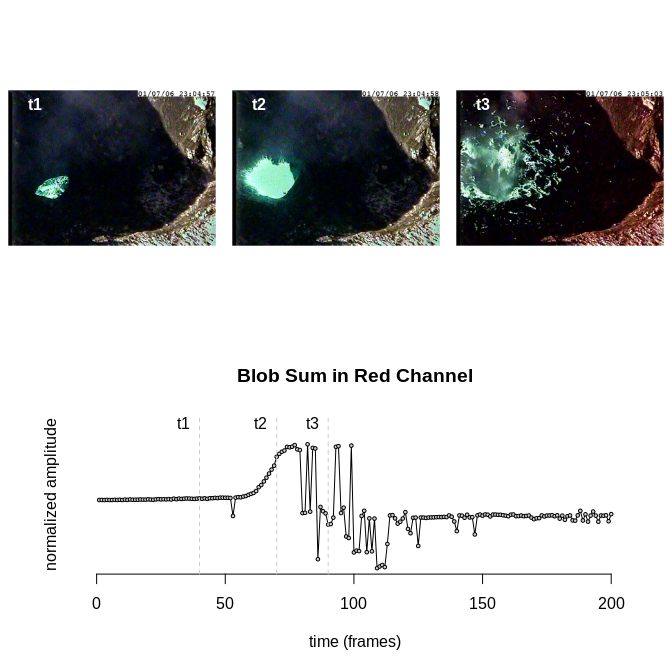## Smooth blob statistics Raw images are often noisy from sudden changes in acquisition settings (e.g. exposure time), camera shaking, instrument noise, etc… Noise may be smoothed by applying a filter in the time domain. ## pick a single statistic from (i.e. the sum of the red channel blob region) blob.sum <- blob.stats$r[,1]

## choose the window length for the running average operator
win.length = 15

## smooth the blob sum stat according to window length
blob.sum.filt <- run.avg(blob.sum,win.length)

## PLOTTING ##
plot(blob.sum,type='o',bg='gray80',xlab="time (frame)",
ylab='blob sum in red channel',pch=21,cex=0.5)
lines(blob.sum.filt,col='gray30',lwd=4)
lines(blob.sum.filt,col='red',lwd=2)

pch=c(21,NA),lty=c(1,1),pt.bg=c('gray30',NA))